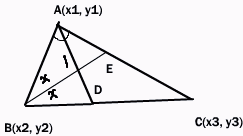Name: ___________________Date:___________________

kwizNET Subscribers, please login to turn off the Ads!
 Email us to get an instant 20% discount on highly effective K-12 Math & English kwizNET Programs!

### High School Mathematics - 29.17 Incentre of a Triangle

 To find the coordinates of the incentre of a triangle whose vertices are given Definition: The point of concurrence of the internal bisectors of the angles of a triangle is called the incentre and is generally denoted by I. Let A(x1, y1), B(x2, y2) and C(x3, y3) be the vertices of the triangle. Let AD and BE, the bisectors of the angles A and B meet in I.AD bisects angleBAC of triangle ABC Hence BD/DC = AB/BC = c/b D divides BC internally in the ratio c:b Hence the coordinates of D are [(cx3+bx2)/(c+b), (cy3 + by2)/(c+b)] Now, BI bisects angle ABD of triangle ABD Hence AI/ID = AB/BD = c/BD----equation 1 But BD/DC = c/b(Proved above) Hence BD/(BD+ DC) = c/(c+b) BD/BC = c/(c+b) Hence BD = ca/(c+b)(because BC = a) From equation 1 AI/ID = c/ca/(c+b) = (c+b)/a This shows that I divides AD internally in the ratio c+b:a The coordinates of I are x = {(c+b)[(cx3+bx2)/(c+b)]+ax1}/(c+b)+a = (ax1+bx2+cx3)/(a+b+c) y = {(c+b)[(cy3+by2)/(c+b)] + ay1}/(c+b) + a = (ay1+by2+cy3)/(a+b+c) Directions: Solve the following.Name: ___________________Date:___________________

### High School Mathematics - 29.17 Incentre of a Triangle

 Q 1: Find the coordinates of the incentre of the triangle whose vertices are (-36,7), (20,7) and (0,-8)(-1,0)(0,1)(1,0)(0,-1) Question 2: This question is available to subscribers only!

#### Subscription to kwizNET Learning System costs less than \$1 per month & offers the following benefits:

• Unrestricted access to grade appropriate lessons, quizzes, & printable worksheets
• Instant scoring of online quizzes
• Progress tracking and award certificates to keep your student motivated
• Unlimited practice with auto-generated 'WIZ MATH' quizzes
• Child-friendly website with no advertisements

© 2003-2007 kwizNET Learning System LLC. All rights reserved. This material may not be reproduced, displayed, modified or distributed without the express prior written permission of the copyright holder. For permission, contact info@kwizNET.com
For unlimited printable worksheets & more, go to http://www.kwizNET.com.